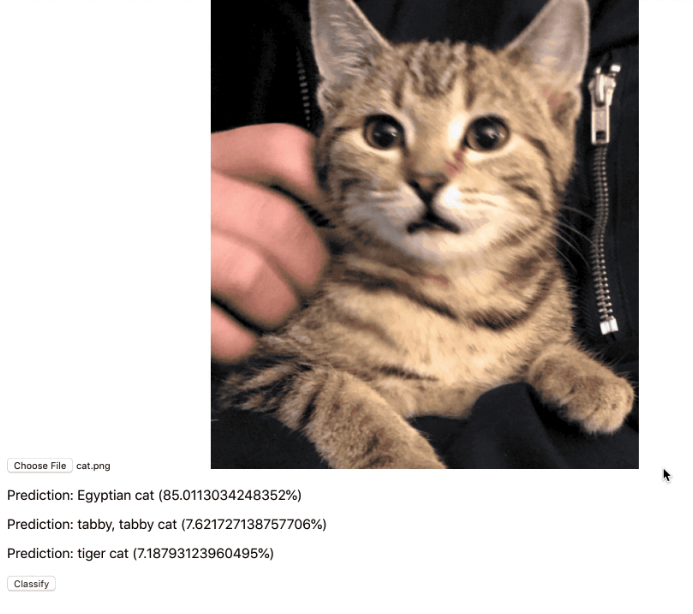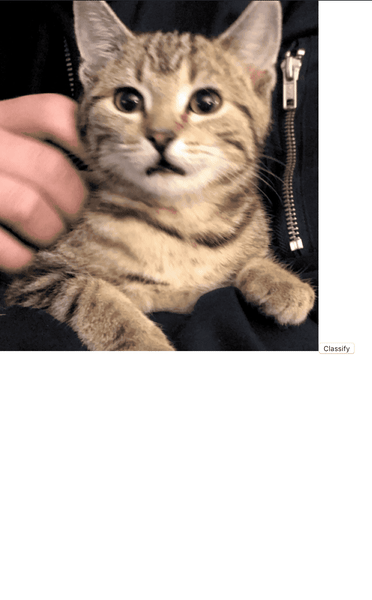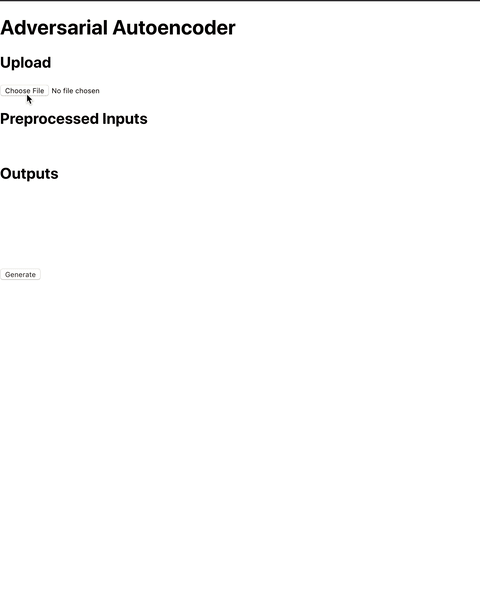# Using a Machine Learning Model in a Web Application

June 30, 2019

This was originally posted to the Tensorpad Blog## Why

Current trends seem to indicate that software engineers will increasingly be asked to apply machine learning models to production software. While the development of the models remain with Data Scientist, trained models often tossed over to Software Engineers. This creates a set of challenges for Software Engineers. Consider a situation where a model needs to be applied to a Web Application. Models are often delivered in Python while the client-side of the Web Application operates on JavaScript. How can Software Engineers apply a Python model on the Web in JavaScript? How could they minimise the data security footprint? Could the model operate offline?

Luckily, it is becoming easier to apply machine learning models on the Web. There are libraries, such as Tensorflow JS which enables the use of models with JavaScript. Additionally, packaging the model with the Web Application client allows the model to operate offline. This also means data does not need to leave the user’s machine for predictions to be made. This is a big data security win :).

## What

We will examine the usage of trained machine learning models in Web Applications. The Web Application will classify images or generate images. This will happen on the client, in JavaScript. We start by learning how to utilize a model in a Web Application. Then we will examine how to prepare our own model. Finally, we will use our own model in a Web Application.

The final code example will be a Web Application which uses a generative neural network. It will use a user uploaded image to generate a new ‘unique’ image.

## Code Repositories

### Web Application

Let’s start by examining how a Web Application can import and interact with a trained machine learning model.

In your local `node.js` environment, perform the following steps:

1. Clone the Web Application’s git repository with: `git clone https://github.com/PeterChauYEG/tfjs-web-app`
2. Navigate to the new directory with: `cd tfjs-web-app`
3. Install it’s dependencies with: `npm install`
4. Start the Web Application with: `npm start`
5. Open your browser to the Web Application: `localhost:3000`

#### Predictions

This demo makes a classification on a known image. Using a known image allows us to quickly verify that the machine learning model had loaded correctly, and works as intended. To do this, we will load MobileNet model and make a classification.

MobileNet is a pretrained machine learning model packaged with Tensorflow JS. It is an Image classifier that was trained using the Imagenet image database. It classifies images into 1 of 1000 classes. It very efficient in terms of speed and size which makes it ideal for use in embedded and mobile applications. All we need to do is load, feed it an input, and interpret it’s output.

1. Checkout the branch called `predictions` with: `git checkout predictions`
2. There should be an image of a cat, and a `Classify` button on `localhost:3000`.
3. Press the `Classify` button. The MobileNet model will be loaded and the image will be classified
4. This classification will be shown as a list of the most likely classes
``````  async classify() {

// Classify the image.
const predictions = await model.classify(this.refs.cat);

this.setState({ predictions })
}``````This demo makes a classification using a user uploaded image. Using a user uploaded allows us to verify that the Web Application can use an abstract image to make a classification. Again, we will use the MobileNet model to make a classification.

1. Checkout the branch called `prediction-uploads` with: `git checkout prediction-uploads`
2. There should be an `Upload` and `Classify` button on `localhost:3000`
3. Upload the image `cat.png` in the `src` directory. The image will be loaded into the Web Application and rendered to the page
4. Press the `Classify` button. The MobileNet model will be loaded and the image will be classified
5. This classification will be shown as a list of the most likely classes
``````  onUpload(e) {
const files = e.target.files
const image = URL.createObjectURL(files)
console.log(files)
this.setState({ image })
}``````#### mnist_aae:

This demo generates an image of a number. It will generate random numbers and feed them into a generative machine learning model. Then the output of the model will be rendered. The output is a generated image based on the MNIST dataset. Using random numbers allows us to verify that the Web Application can load a custom generative machine learning model, and generate images with it.

The machine learning model is half of an adversarial autoencoder. These types of models will be discussed in a later section.

1. Checkout the branch called `mnist_aae` with: `git checkout mnist_aae`
2. There should be a `Generate` button on `localhost:3000`
3. Press the `Generate` button. The Web Application will load the model, generate random numbers, feed them into the model, process the output into pixels, and render them onto the page.
4. The generated random numbers will be shown
5. The generated image will be shown
``````  async generate() {

// input
const input = tf.randomNormal([1,10])
const inputValues = await input.data()
this.setState({ input: Array.prototype.slice.call(inputValues) })

// Classify the image.
const generated = await model.predict(input);
const reshapedGenerated = generated.reshape([28, 28])
let normalizedGenerated = reshapedGenerated.sub(-1)
normalizedGenerated = normalizedGenerated.div(2)

await tf.browser.toPixels(normalizedGenerated, this.refs.canvas);
}``````This demo uses a user upload to generate an image of a number. It will use the file’s hash to generate random numbers. These random numbers will be feed into a generative machine learning model. Then the output of the model will be rendered. Using the upload file’s hash allows us to verify that we can use an abstract image to generate a image. Again, we will use half of an adversarial autoencoder.

1. Checkout the branch called `mnist_aae_upload` with: `git checkout mnist_aae_upload`
2. There should be a file upload button
3. Upload the image `cat.png` in the `src` directory. The Web Application will generate random numbers, load the model, generate an output, process the output into pixels, and render them onto the page.
4. The hash will be shown
5. The randomly generated numbers will be shown
6. The generated image will be shown
``````  async generate() {

// input
let totalHash = 0
const splitHash = this.state.hash.split('-')
splitHash
.filter(i => i !== '-')
.forEach(i => {
const parsedInt = parseInt(i, 16)
totalHash =+ parsedInt
})

const input = tf.randomNormal([1,10], undefined, undefined, undefined, totalHash)
const inputValues = await input.data()

this.setState({ input: Array.prototype.slice.call(inputValues), seed: totalHash })

// Classify the image.
const generated = await model.predict(input);
const reshapedGenerated = generated.reshape([28, 28])
let normalizedGenerated = reshapedGenerated.sub(-1)
normalizedGenerated = normalizedGenerated.div(2)

await tf.browser.toPixels(normalizedGenerated, this.refs.canvas);
}

const files = e.target.files
const image = URL.createObjectURL(files)
const hash = image.split('blob:http://localhost:3000/')
this.setState({ hash })
this.generate()
}``````This demo also uses a user upload to generate an image of a number. However we use a different method of converting the image into an input. Instead of using the file’s hash, we will feed in a preprocessed version of the image. This process is an estimation of the adversarial autoencoder’s encoder half. It transforms the image into the format the machine learning model expects. After it is fed into the model, the output will be rendered.

1. Checkout the branch called `mnist_aae_upload_from_image` with: `git checkout mnist_aae_upload_from_image`
2. There should be a file upload button and a `Generate` button
3. Upload the image `cat.png` in the `src` directory. The image will be loaded into the Web Application.
4. Press the generate button. The Web Application will load the model, the image will be preprocessed, generate an output, process the output into pixels, and render them onto the page
5. The preprocessed imaged will shown.
6. The generated image will be shown.
``````    async generate() {

// input
const input = tf.browser.fromPixels(this.refs.image).asType('float32')
const resizedInput = tf.image.resizeNearestNeighbor(input, [1, 10]).div(255)
const grayScaledInput = tf.mean(resizedInput, 2, )

await tf.browser.toPixels(grayScaledInput, this.refs.inputCanvas);

// Classify the image.
const decoded = await generator.predict(grayScaledInput);
const reshapedGenerated = decoded.reshape([28, 28])
let normalizedGenerated = reshapedGenerated.sub(-1)
normalizedGenerated = normalizedGenerated.div(2)

await tf.browser.toPixels(normalizedGenerated, this.refs.canvas);
}

const files = e.target.files
const image = URL.createObjectURL(files)
this.setState({ image })
}``````## What is an adversarial autoencoder?

An adversarial autoencoder is defined as:

“a probabilistic autoencoder that uses the recently proposed generative adversarial networks (GAN) to perform variational inference by matching the aggregated posterior of the hidden code vector of the autoencoder with an arbitrary prior distribution. Matching the aggregated posterior to the prior ensures that generating from any part of prior space results in meaningful samples. As a result, the decoder of the adversarial autoencoder learns a deep generative model that maps the imposed prior to the data distribution.” - https://arxiv.org/abs/1511.05644

An adversarial autoencoder takes in images and encodes them down into a relatively small dimensions. Then it decodes them into the original image. The first half is the encoder, and the second half is the decoder. When this type of machine learning model is trained, it is learning to compress and decompress images without loss. The adversarial part refers to an additional technique which allows the network to learn better. See this link for a better overview.

When we only use the decoder. It’s input is some small set of numbers. The decoder will try to construct this into an image that looks like what the full machine learning model was trained with.

## Repurposing a model

Adversarial autoencoders can be used to create a new image out of ‘static’. To do this cut the adversarial autoencoder in half and use the decoder half to generate new images. Lets examine how to do this.

### Environment

It is common to use Jupyter Notebooks to when working with machine learning models. I typically use a web based notebook. It allows me to work on the model from any machine and leverage cloud GPUs. Lets use one such solution, Tensorpad.

1. Create a Tensorpad account. Apply promo code `generativemodel` on the billing page for 5 free hours of GPU compute time.
2. `Create JupyterLab Job` with `TensorFlow 1.11`. Wait for the instance to be created then `Open Lab`.
3. Open a `Terminal` in the opened lab.

### Model Setup

First we need a generative machine learning model. I used this adversarial autoencoder model

1. Clone it’s Github repository with: `\$ git clone https://github.com/eriklindernoren/Keras-GAN.git`
2. Create a new notebook called `model`
3. Create the directories `images` and `saved_model`
4. Open `model`
5. Copy the contents of `Kera-GAN/aae/aae.py` into it `model`
6. Modify the model to get it running with the current TF API:

`from keras.layers import merge` -> `from keras.layers import Lam`

``````    latent_repr = merge([mu, log_var],
mode=lambda p: p + K.random_normal(K.shape(p)) * K.exp(p / 2),
output_shape=lambda p: p)``````

->

``````    latent_repr = Lambda(
lambda p: p + K.random_normal(K.shape(p)) * K.exp(p / 2),
output_shape=lambda p: p
)([mu, log_var])``````

### Exporting the model

To load the machine learning model into Tensorflow.js, we need to convert the `python` model. There are many different model formats. Tensorflow.js uses `.h5`.

1. Modify the `save_model` function:
``````def save_model(self):
def save(model, model_name):
model_path = "saved_model/%s.json" % model_name
weights_path = "saved_model/%s_weights.hdf5" % model_name
options = {"file_arch": model_path,
"file_weight": weights_path}
json_string = model.to_json()
open(options['file_arch'], 'w').write(json_string)
model.save_weights(options['file_weight'])

save(self.generator, "aae_generator")
save(self.discriminator, "aae_discriminator")``````

->

``````def save_model(self):

def save(model, model_name):
model_path = "saved_model/%s.json" % model_name
weights_path = "saved_model/%s_weights.hdf5" % model_name
options = {"file_arch": model_path,
"file_weight": weights_path}
json_string = model.to_json()
open(options['file_arch'], 'w').write(json_string)
model.save_weights(options['file_weight'])

save(self.decoder, "aae_generator")
self.decoder.save('saved_model/aae_generator.h5')``````
1. Run the whole notebook by using the `Run` menu and selecting `Run All Cells`. Running the notebook will generate files for the `aae_generator` called `aae_generator.h5` in the `saved_model` dir.
2. Place these files in the Web Application’s `public` dir.
3. Run `yarn convert:generator` in the Web Application’s root dir will create a folder in the `public` dir called `generator`
4. The outputted directory contains the model files you need to use with `tensorflow.js`

#### NOTE

`yarn convert:generator` calls `tensorflowjs_converter --input_format keras public/aae_generator.h5 public/generator`. You may need to install `tensorflowjs_converter` with: `\$ pip install tensorflowjs`. If you have any issues, then you likely have an issue with your `python` environment. I used `conda` to create an environment with `python 2.7.16`. There are other dependencies you may need to install but that is outside the scope of this guide. Message me if you’d like some help.

## Next Steps

Where can you go from here? Try to:

1. Retrain the adversarial autoencoder with ImageNet or a custom dataset to generate more classes of images
2. Use the encoder model to encode the uploaded image so that the output is better

## What can be done?Written by Peter Chau, a Canadian Software Engineer building AIs, APIs, UIs, and robots.